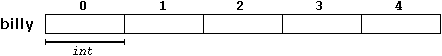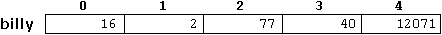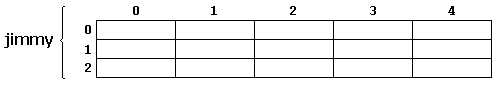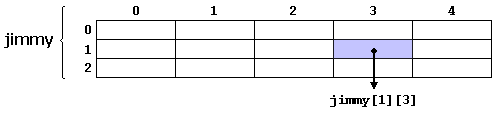# C++ 数组type name [elements];

int billy ;

## 初始化数组(Initializing arrays)

int billy ;int billy  = { 16, 2, 77, 40, 12071 };int billy [] = { 16, 2, 77, 40, 12071 };

name[index]billy = 75;

a = billy;

int billy; // 声明新数组(以数据类型名称开头)
billy = 75; // 存储数组的一个元素

billy = a; // a为一个整型变量
billy[a] = 75;
b = billy [a+2];
billy[billy[a]] = billy + 5;

// arrays example
#include <iostream.h>

int billy [ ] = {16, 2, 77, 40, 12071};
int n, result=0;

int main () {
for ( n=0 ; n<5 ; n++ ) {
result += billy[n];
}

cout << result;
return 0;
}

12206

## 多维数组（Multidimensional Arrays）jimmy 显示了一个整型(int )的3x5二维数组，声明这一数组的的方式是：

int jimmy ;(记住数组的索引总是从0开始)。

char century ;

int jimmy ; 效果上等价于
int jimmy ; (3 * 5 = 15)

 // multidimensional array #include #define WIDTH 5 #define HEIGHT 3 int jimmy [HEIGHT][WIDTH]; int n,m; int main (){ for (n=0; n #define WIDTH 5 #define HEIGHT 3 int jimmy [HEIGHT * WIDTH]; int n,m; int main (){ for (n=0; n#define HEIGHT 3

#define HEIGHT 4

### 数组参数（Arrays as parameters）

void procedure (int arg[])

int myarray ;

procedure (myarray);

// arrays as parameters
#include <iostream.h>

void printarray (int arg[ ], int length) {
for (int n=0; n<length; n++) {
cout << arg[n] << " ";
}
cout << "\n";
}

int main () {
int firstarray[ ] = {5, 10, 15};
int secondarray[ ] = {2, 4, 6, 8, 10};
printarray (firstarray,3);
printarray (secondarray,5);
return 0;
}

5 10 15
2 4 6 8 10

base_type[ ][depth][depth]

void procedure (int myarray[ ])